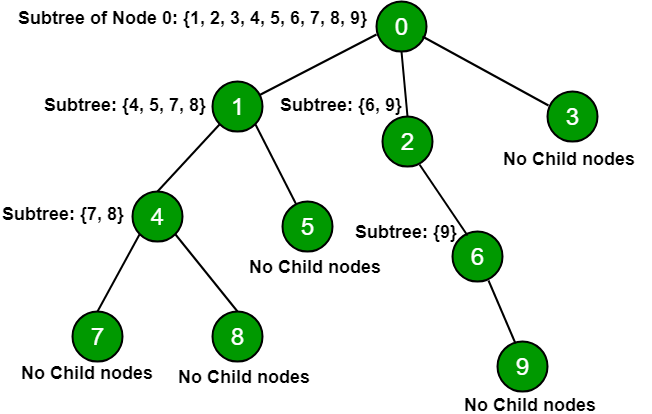# Subtree of all nodes in a tree using DFS

Given n nodes of a tree and their connections, print Subtree nodes of every node.

Subtree of a node is defined as a tree which is a child of a node. The name emphasizes that everything which is a descendant of a tree node is a tree too, and is a subset of the larger tree.Examples :

```Input: N = 5
0 1
1 2
0 3
3 4
Output:
Subtree of node 0 is 1 2 3 4
Subtree of node 1 is 2
Subtree of node 3 is 4

Input: N = 7
0 1
1 2
2 3
0 4
4 5
4 6
Output:
Subtree of node 0 is 1 2 3 4 5 6
Subtree of node 1 is 2 3
Subtree of node 4 is 5 6
```

## Recommended: Please try your approach on {IDE} first, before moving on to the solution.

Approach: Do DFS traversal for every node and print all the nodes which are reachable from a particular node.
Explanation of below code:

1. When function dfs(0, 0) is called, start = 0, dfs_order.push_back(0), visited = 1 to keep track of dfs order.
2. Now, consider adjacency list (adj) as considering directional path elements connected to node 0 will be in adjacency list corresponding to node 0.
3. Now, recursively call dfs function till all elements traversed of adj.
4. Now, dfs(1, 2) is called, Now start = 1, dfs_order.push_back(1), visited = 1 after adj elements is traversed.
5. Now adj  is traversed which contain only node 2 when adj is traversed it contains no element, it will break and end=2.
6. Similarly, all nodes traversed and store dfs_order in array to find subtree of nodes.

## C++

 `// C++ code to print subtree of all nodes ` `#include ` `using` `namespace` `std; ` ` `  `// arrays for keeping position ` `// at each dfs traversal for each node ` `int` `start; ` `int` `endd; ` ` `  `// Storing dfs order ` `vector<``int``>dfs_order; ` `vector<``int``>adj; ` `int` `visited; ` ` `  `// Recursive function for dfs ` `// traversal dfsUtil() ` `void` `dfs(``int` `a,``int` `&b) ` `{ ` ` `  `    ``// keep track of node visited ` `    ``visited[a]=1; ` `    ``b++; ` `    ``start[a]=b; ` `    ``dfs_order.push_back(a); ` `     `  `    ``for``(vector<``int``>:: iterator it=adj[a].begin(); ` `                           ``it!=adj[a].end();it++) ` `    ``{ ` `        ``if``(!visited[*it]) ` `        ``{ ` `            ``dfs(*it,b); ` `        ``} ` `    ``} ` `    ``endd[a]=b; ` `} ` ` `  `// Function to print the subtree nodes ` `void` `Print(``int` `n) ` `{ ` `    ``for``(``int` `i = 0; i < n; i++) ` `    ``{ ` `        ``// if node is leaf node ` `        ``// start[i] is equals to endd[i] ` `        ``if``(start[i]!=endd[i]) ` `        ``{ ` `            ``cout<<``"subtree of node "``<

## Python3

 `# Python3 code to print subtree of all nodes  ` ` `  `# arrays for keeping position at  ` `# each dfs traversal for each node  ` `start ``=` `[``None``] ``*` `100001`  `endd ``=` `[``None``] ``*` `100001` ` `  `# Storing dfs order  ` `dfs_order ``=` `[]  ` `adj ``=` `[[] ``for` `i ``in` `range``(``100001``)]  ` `visited ``=` `[``False``] ``*` `100001` ` `  `# Recursive function for dfs traversal dfsUtil()  ` `def` `dfs(a, b):  ` `  `  `    ``# keep track of node visited  ` `    ``visited[a] ``=` `1`  `    ``b ``+``=` `1` `    ``start[a] ``=` `b  ` `    ``dfs_order.append(a)  ` `     `  `    ``for` `it ``in` `adj[a]:  ` `        ``if` `not` `visited[it]:  ` `            ``b ``=` `dfs(it, b)  ` `      `  `    ``endd[a] ``=` `b ` `    ``return` `b ` `  `  `# Function to print the subtree nodes  ` `def` `Print``(n):  ` `  `  `    ``for` `i ``in` `range``(``0``, n):  ` `      `  `        ``# If node is leaf node  ` `        ``# start[i] is equals to endd[i]  ` `        ``if` `start[i] !``=` `endd[i]:  ` `          `  `            ``print``(``"subtree of node"``, i, ``"is"``, end ``=` `" "``)  ` `            ``for` `j ``in` `range``(start[i]``+``1``, endd[i]``+``1``):  ` `              `  `                ``print``(dfs_order[j``-``1``], end ``=` `" "``)  ` `              `  `            ``print``() ` ` `  `# Driver code  ` `if` `__name__ ``=``=` `"__main__"``:  ` `  `  `    ``# No of nodes n = 10  ` `    ``n, c ``=` `10``, ``0`  `     `  `    ``adj[``0``].append(``1``)  ` `    ``adj[``0``].append(``2``)  ` `    ``adj[``0``].append(``3``)  ` `    ``adj[``1``].append(``4``)  ` `    ``adj[``1``].append(``5``)  ` `    ``adj[``4``].append(``7``)  ` `    ``adj[``4``].append(``8``)  ` `    ``adj[``2``].append(``6``)  ` `    ``adj[``6``].append(``9``)  ` `     `  `    ``# Calling dfs for node 0  ` `    ``# Considering root node at 0  ` `    ``dfs(``0``, c)  ` ` `  `    ``# Print child nodes  ` `    ``Print``(n) ` `  `  `# This code is contributed by Rituraj Jain `

Output:

```subtree of node 0 is 1 4 7 8 5 2 6 9 3
subtree of node 1 is 4 7 8 5
subtree of node 2 is 6 9
subtree of node 4 is 7 8
subtree of node 6 is 9
```

My Personal Notes arrow_drop_upCheck out this Author's contributed articles.

If you like GeeksforGeeks and would like to contribute, you can also write an article using contribute.geeksforgeeks.org or mail your article to contribute@geeksforgeeks.org. See your article appearing on the GeeksforGeeks main page and help other Geeks.

Please Improve this article if you find anything incorrect by clicking on the "Improve Article" button below.

Improved By : rituraj_jain